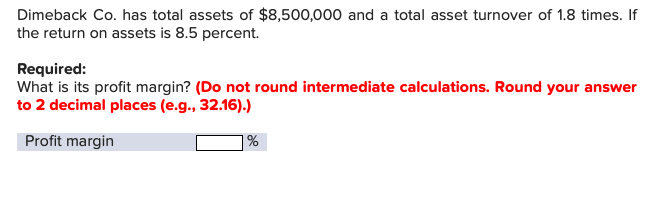# Dimeback Co. has total assets of $8,500,000 and a total asset turnover of 1.8 times. If the return on assets is 8.5 percent. Required: What is its profit margin? (Do not round intermediate calculations. Round your answer to 2 decimal places (e.g., 32.16).) Profit margin Questionhelp_outlineImage TranscriptioncloseDimeback Co. has total assets of$8,500,000 and a total asset turnover of 1.8 times. If the return on assets is 8.5 percent. Required: What is its profit margin? (Do not round intermediate calculations. Round your answer to 2 decimal places (e.g., 32.16).) Profit margin fullscreen

### Want to see this answer and more?

Experts are waiting 24/7 to provide step-by-step solutions in as fast as 30 minutes!*

*Response times vary by subject and question complexity. Median response time is 34 minutes and may be longer for new subjects.
Tagged in